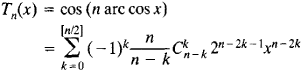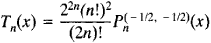# Chebyshev Polynomials

Also found in: Wikipedia.

## Chebyshev polynomials

[′cheb·ə·shəf ‚päl·i′nō·mē·əlz]
(mathematics)
A family of orthogonal polynomials which solve Chebyshev's differential equation.
McGraw-Hill Dictionary of Scientific & Technical Terms, 6E, Copyright © 2003 by The McGraw-Hill Companies, Inc.
The following article is from The Great Soviet Encyclopedia (1979). It might be outdated or ideologically biased.

## Chebyshev Polynomials

Chebyshev polynomials of the first kind are a special system of polynomials of successively increasing degree. For n = 0, 1, 2, . . . they are defined by the formulaIn particular, T0 = 1, T1 = x, T2 = 2x2 – 1, T3 = 4x3 – 3x and T4 = 8x4 – 8x2 + 1.

The polynomials Tn (x) are orthogonal with respect to the weight function (1 – x2)–½ on the interval [–1, +1] (seeORTHOGONAL POLYNOMIALS). They satisfy the differential equation

(1 – x2)yxy + n2y = 0

and the recursion formula

Tn+1 (x) = 2xTn(x) – Tn – 1(x)

Chebyshev polynomials of the first kind are a special case of the Jacobi polynomials Pn(α,β)(x):Chebyshev polynomials of the second kind Un (x) are a system of polynomials that are orthogonal with respect to the weight function (1 – x2)½ on the interval [–1, +1]. The relation between Chebyshev polynomials of the second kind and Chebyshev polynomials of the first kind is given by, for example, the recursion formula

(1 – x2)Un – 1 (x) = xTn (x) – Tn+l(x)

### REFERENCES

Chebyshev, P. L. Poln. sobr. soch., vols. 2–3. Moscow-Leningrad, 1947–48.
Szegö, G. Ortogonal’nye mnogochleny. Moscow, 1962. (Translated from English.)
References in periodicals archive ?
Applying the Chebyshev polynomials of orthogonality feature [7, 8], we get an infinite system of algebraic equations respectively to the searched solution:
To use the same idea, we cannot use the bounds of Theorem 1.1 but have to resort to a slightly different approach: Instead of using a polynomial approximation for the inverse directly, we use shifted and normalized Chebyshev polynomials similarly to what was done in  for general line segments in the complex plane.
Taking into account the considered assumptions the solution of equation (9) can be obtained by means of the Chebyshev expansion of the left side and the known spectral relations for the Chebyshev polynomials (Appendix).
where [T.sup.*.sub.k](x) is used here to express the usual Chebyshev polynomials of the first kind; the independent variable x is defined on [-1, 1]; and v is a parameter which refers to the number of the truncated coefficients.
To estimate the time derivative term in the unsteady flow governing equations, the time-marching method employs a finite difference method, while time-spectral methods used in this study apply a discrete Fourier expansion and Chebyshev polynomials.
We may approximate the function f on the interval [-1, 1] by using Chebyshev polynomials  of the first kind.
The Chebyshev polynomials are used to solve composition FOCPs and Chebyshev polynomialsTn(f) of degree n are important in approximation theory, since the roots of the Chebyshev polynomials of the first kind, which are also called Chebyshev nodes, are used as nodes in polynomial interpolation.
Hosseini, "Adomian decomposition method with Chebyshev polynomials," Applied Mathematics and Computation, vol.
This model is explored subject to both Dirichlet and Neumann boundary conditions on the bounded domain [OMEGA] = [-1, 1] x [-1, 1] to satisfy the domain required by the Chebyshev polynomials with initial condition w(x, y, 0) = f(x, y).
In recent years, various numerical methods have been proposed for solving fractional diffusion equations, these methods include wavelets methods [11-17], Jacobi, Legendre, and Chebyshev polynomials methods [18-21], spectral methods [22, 23], finite element method , wavelet Galerkin method , and finite difference methods [26, 27].
The computational cost of extended Chebyshev polynomials is lower, compared to the traditional modular exponentiation operation and the point addition operation of elliptic curve [20-24].
One of the most important properties is that Chebyshev polynomials are the so-called semi-group property which establishes that

Site: Follow: Share:
Open / Close